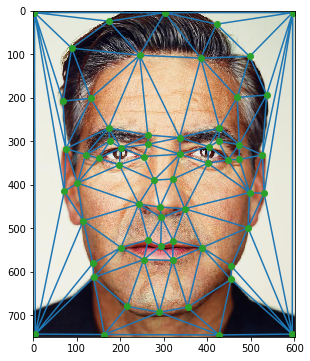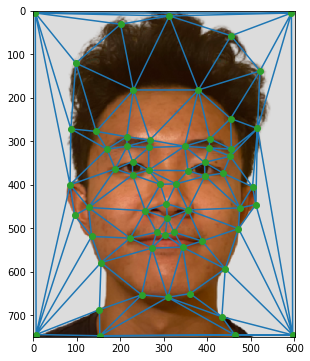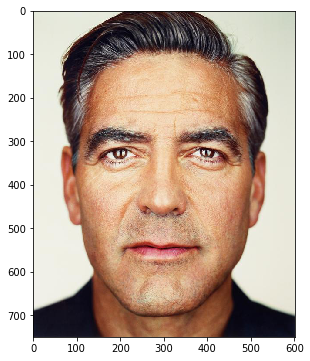# CS-194 Project 3¶

By: Calvin Chen

For this project, I worked on morphing faces, particularly mines to George Clooney's, computed the mean face for a variety of Danish people, and extrapolated from that population mean to create caricatures of myself.

# Defining Correspondence¶

For this section, I collected points for my face and George Clooney's in order to find a correspondence between facial features, and constructed a Delaunay Triangulation on the average face.

### Faces, Points, and Triangulations¶

George Clooney Triangulation and PointsMy Triangulation and Points# Computing the "Mid-Way Face"¶

For this section, I broke down the problem into the following algorithm.

### Algorithm:¶

1. Find the midway shape between the two averages by taking the average of the two sets of feature points and compute the Delaunay Triangulation on that.
2. Take the simplices, or indices of triangles, from the result and extract the estimated coordinates from the original images.
3. Create an interpolation for each of the original images over all 3 colors channels (RGB). This way, when translating points from the mid-way face back to the original ones, we're able to better estimate RGB values even if the translations don't end up at exact pixel coordinates.
4. For each of the triangles, calculate the affine transformation between the midway face and the original face.
5. For each of the triangles, map the pixels within each triangle back to the original images using the affine transformation matrix for that specific triangle, and use the interpolation functions returned for RGB to determine what pixel value should exist in the mid-way shape for that location.
6. Take an average of the two values from both images for each color channel.
7. Output the mid-way shape with its averaged colors.

George Clooney's FaceMidway Image